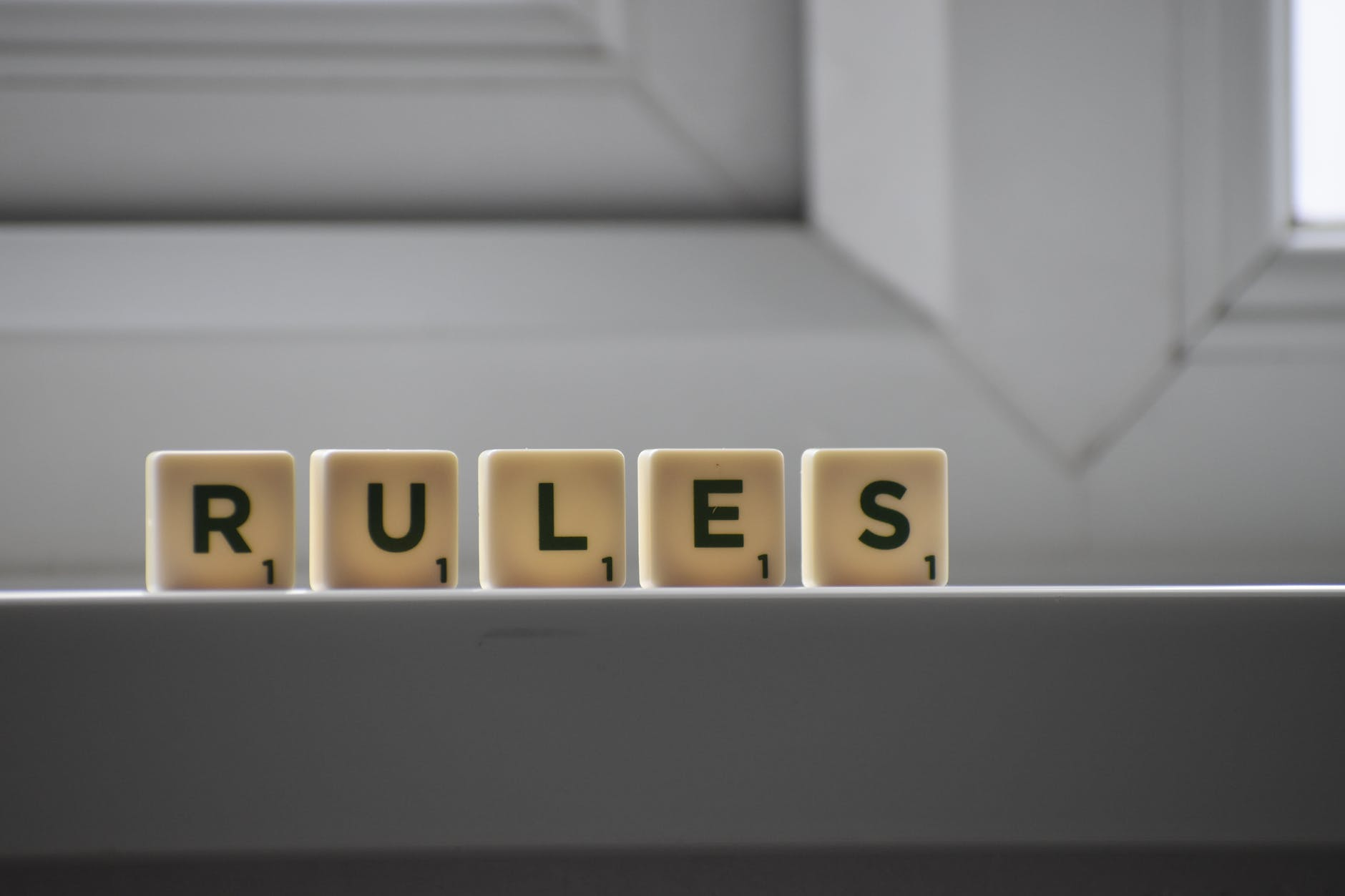For Next Loops in Excel VBA

For Next loops are a type of count controlled loop in VBA. By count controlled it is meant that this loop is performed a certain number of times as determine by the criteria set by the developer. In this post, we are going to learn how to use For Next loops with functions and with Subroutines.

For Next Explained

Below is a high level overview of the behavior of For Next loops in a visual.

The For Next loop begins at i. This is normally one but it is flexible. In addition, the value normally increases by 1 also. Next, we have the loop it repeats until i reaches whatever the limit is. Often the limit is set by the number of rows or some other means and is generally called n in coding. The limit continues based on an if then statement. If i is less than the limit then it will increase by one and repeat the process. If i is greater than the limit then it will exit.

One thing that is missing from this diagram that is also important is some sort of way to count how many times the For Next loop finds what the developer is looking for. This value is often set to c and increase by one each time through the code. This will make more sense in an actual example.

Code for Function

Below is the code for a function that uses a For Next loop. This function will check if out number is divisible by 4 or 7.

Function numberCheck(n As Integer) As Integer
Dim i As Integer, c As Integer
For i = 1 To n
If i Mod 4 = 0 Or i Mod 7 = 0 Then
c = c + 1
End If
Next i
numberCheck = c
End Function

In the code above we are doing the following.

1. Creating a function called “numberCheck) that sets n as our variable for the limit
2. We dim the c and c variables
3. Next, is our loop, i is set to 1 and will continue until n which is picked by the developer
4. After this, is our If Then statement. If our number from n is divisible by 4 or 7 then the c value will go up 1.
5. Then we repeat this process until we reach the end of n
6. Exit function.

The image below shows this function in action.

The function is counting how many numbers between 1 and 16 are divisible by 4 or 7. The answer is 6.

Subroutine Code

We will now complete a similiar task with a subroutine. This time we want to count a selection of rows and see how many number 3s it has. Below is the code.

Sub CountThrees()
Dim nr As Integer, i As Integer, c As Integer
nr = Selection.Rows.Count
For i = 1 To nr
If Selection.Cells(i, 1) = 3 Then c = c + 1
Next i
MsgBox ("There are " & c & " threes in your selection.")
End Sub

This code does the following

1. We give the subroutine the name “CountThrees”
2. We dim nr for the number of rows, and we also dim i and c as in the previous example
3. The for next loop is next and goes from 1 to the end of all the rows
4. Inside the for next loop the if then statement looks at the cells that are selected in the Excel spreadsheet and searches for those that are the number for. Then these 3 are counted and saved in the c function
5. Then the process repeats it’s self
6. At the end, a message bx appears telling the user how many threes are found

Below is an image of this codes actions

Here is the data the subroutine will analyze. You can count for yourself that there are three 3 in this column

Below is the result

The image below shows what is happening in VBA

The image above comes from the locals window in VBA. You can see at the end of the four loop that we ten rows of data (nr) i was set to 11, which is greater than nr which cause the loop to exit. Lastly, c was set to three which indicates that there were 3 threes in the selection

Conclusion

For Next loops are another useful tool for VBA developers. They allow you to let the context of the code determine how many iterations the loop completes.

1 thought on “For Next Loops in Excel VBA”

1.Math Sux